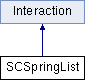ParM  parm A molecular dynamics library
SCSpringList Class Reference

#include <interaction.hpp>

Inheritance diagram for SCSpringList:Public Member Functions

SCSpringList (SCAtomVec *scs, flt eps, flt sig, flt l)
Create an SCSpringList based on scs, using an epsilon of eps, a diameter of sigma, and a "length" of l, where l is cap center-to-cap center distance. More...

SCSpringList (SCAtomVec *scs, flt eps, flt sig, vector< flt > ls)

flt energy (Box &box)
Potential energy due to this Interaction. More...

void set_forces (Box &box)

flt set_forces_get_pressure (Box &box)
Set forces (Atom.f) and return $$P = \sum_{\left<i,j \right>} \vec r_{ij} \cdot \vec F_{ij}$$ at the same time (see pressure()). More...

flt pressure (Box &box)
Partial pressure due to this Interaction. More...

Matrix set_forces_get_stress (Box &box)

Matrix stress (Box &box)
The force-moment tensor for the current simulation: More...

void ignore (uint n1, uint n2)

flt volume ()

flt phi (Box &box)

void ignore (AtomID a1, AtomID a2)

~SCSpringList ()Public Member Functions inherited from Interaction
virtual ~Interaction ()

Constructor & Destructor Documentation

 SCSpringList::SCSpringList ( SCAtomVec * scs, flt eps, flt sig, flt l )
inline

Create an SCSpringList based on scs, using an epsilon of eps, a diameter of sigma, and a "length" of l, where l is cap center-to-cap center distance.

 SCSpringList::SCSpringList ( SCAtomVec * scs, flt eps, flt sig, vector< flt > ls )
inline
 SCSpringList::~SCSpringList ( )
inline

Member Function Documentation

 flt SCSpringList::energy ( Box & box )
virtual

Potential energy due to this Interaction.

Implements Interaction.

 void SCSpringList::ignore ( uint n1, uint n2 )
inline
 void SCSpringList::ignore ( AtomID a1, AtomID a2 )
inline
 flt SCSpringList::phi ( Box & box )
inline
 flt SCSpringList::pressure ( Box & box )
virtual

Partial pressure due to this Interaction.

$$P = \sum_{\left<i,j \right>} \vec r_{ij} \cdot \vec F_{ij}$$, or equivalently $$P = \sum_i \vec r_i \cdot \vec F_i$$

Note that the full pressure involves all interactions and temperature, and needs to be normalized by $$\frac{1}{dV}$$ where $$d$$ is the number of dimensions and $$V$$ is the volume.

Implements Interaction.

 void SCSpringList::set_forces ( Box & box )
virtual

Implements Interaction.

 flt SCSpringList::set_forces_get_pressure ( Box & box )
virtual

Set forces (Atom.f) and return $$P = \sum_{\left<i,j \right>} \vec r_{ij} \cdot \vec F_{ij}$$ at the same time (see pressure()).

Reimplemented from Interaction.

 Matrix SCSpringList::set_forces_get_stress ( Box & box )
 Matrix SCSpringList::stress ( Box & box )
virtual

The force-moment tensor for the current simulation:

$$\tau^{\alpha \beta} = \sum_{\left<i,j \right>} r_{ij}^\alpha F_{ij}^\beta$$.

At $$T=0$$, the stress tensor $$\Sigma = \frac{1}{dV} \tau$$.

Reimplemented from Interaction.

 flt SCSpringList::volume ( )

The documentation for this class was generated from the following files: